# 关于分类数据编码所需了解的所有信息（使用Python代码）

### 总览

• 了解什么是分类数据编码

• 了解不同的编码技术以及何时使用它们

### 介绍• 什么是分类数据？
• 标签编码或有序编码
• 独热编码
• 虚拟编码
• 效果编码
• 二进制编码
• BaseN编码
• 哈希编码
• 目标编码

### 什么是分类数据？

1. 一个人居住的城市：德里，孟买，艾哈迈达巴德，班加罗尔等。
2. 一个人工作的部门：财务，人力资源，生产部。
3. 一个人拥有的最高学位：高中，学士，硕士，博士学位。
4. 学生的成绩：A +，A，B +，B，B-等。

pip install category_encoders

### 标签编码或有序编码

import category_encoders as ce
import pandas as pd
train_df=pd.DataFrame({'Degree':['High school','Masters','Diploma','Bachelors','Bachelors','Masters','Phd','High school','High school']})

# 创建Ordinalencoding的对象
encoder= ce.OrdinalEncoder(cols=['Degree'],return_df=True,
mapping=[{'col':'Degree',
'mapping':{'None':0,'High school':1,'Diploma':2,'Bachelors':3,'Masters':4,'phd':5}}])

#原始数据
train_df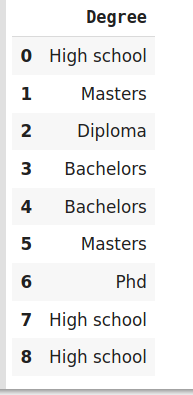# 调整并转换数据
df_train_transformed = encoder.fit_transform(train_df)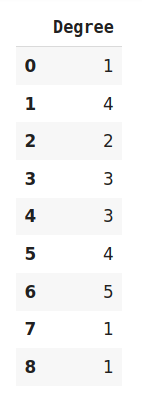### 独热编码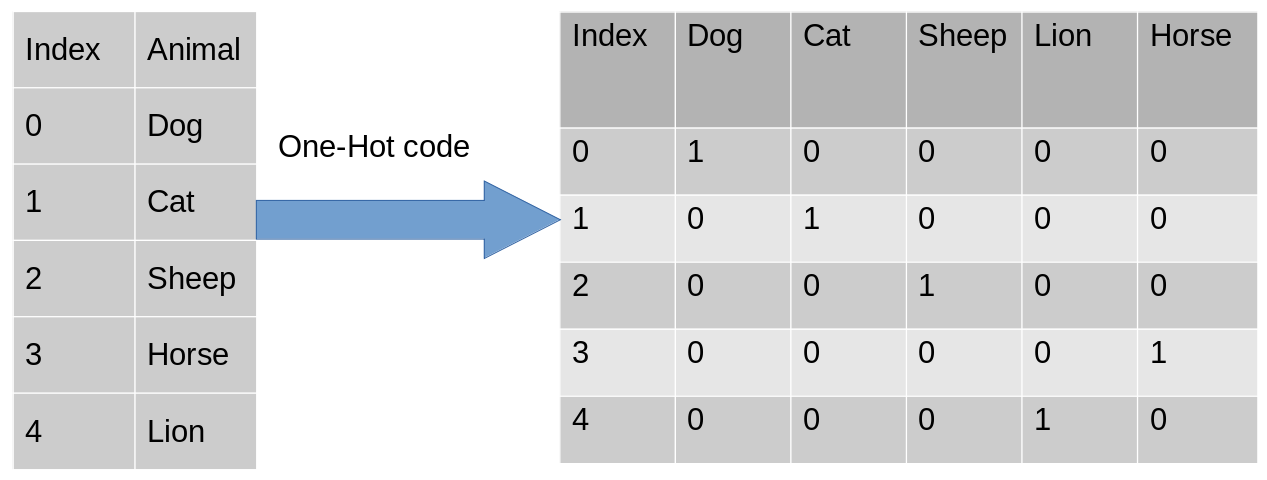import category_encoders as ce
import pandas as pd
data=pd.DataFrame({'City':[
]})

#创建用于独热编码的对象
encoder=ce.OneHotEncoder(cols='City',handle_unknown='return_nan',return_df=True,use_cat_names=True)

# 原始数据
data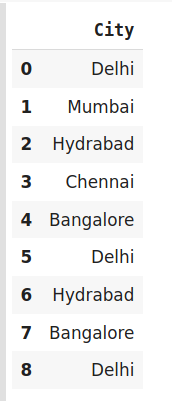# 调整和转换数据
data_encoded = encoder.fit_transform(data)
data_encoded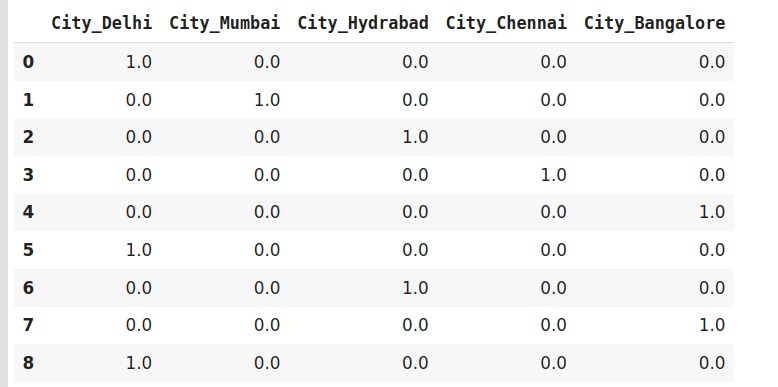### 虚拟编码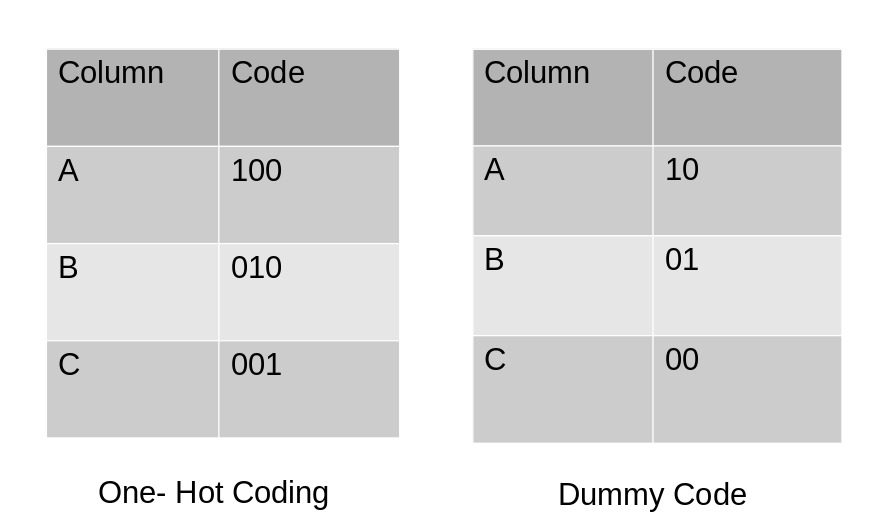import category_encoders as ce
import pandas as pd

# 原始数据
data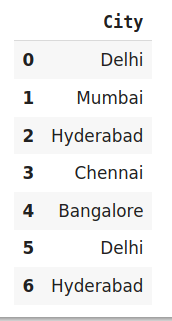#编码数据
data_encoded=pd.get_dummies(data=data,drop_first=True)
data_encoded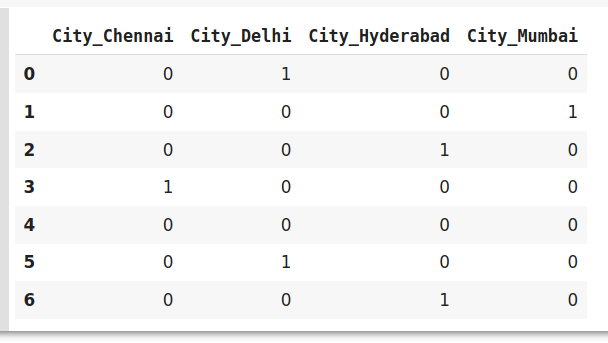#### 独热和虚拟编码的缺点

1. 数据中存在大量级别。在这种情况下，如果一个特征变量中有多个类别，则我们需要相似数量的虚拟变量来对数据进行编码。例如，具有30个不同值的列将需要30个新变量进行编码。

2. 如果我们在数据集中具有多个分类特征，则将发生类似的情况，并且我们最终会有几个二进制特征，每一个都代表分类特征和它们的多个类别，例如一个包含10个或更多分类列的数据集。

### 效果编码（Effect Encoding）

import category_encoders as ce
import pandas as pd

# 原始数据
dataencoder.fit_transform(data)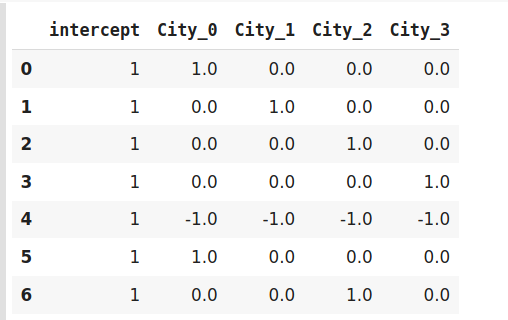### 哈希编码器

import category_encoders as ce
import pandas as pd

#Create the dataframe
data=pd.DataFrame({'Month':['January','April','March','April','Februay','June','July','June','September']})

#Create object for hash encoder
encoder=ce.HashingEncoder(cols='Month',n_components=6)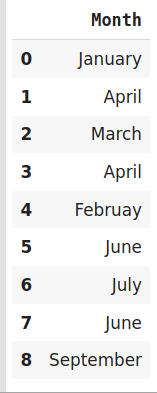# 调整和转换数据
encoder.fit_transform(data)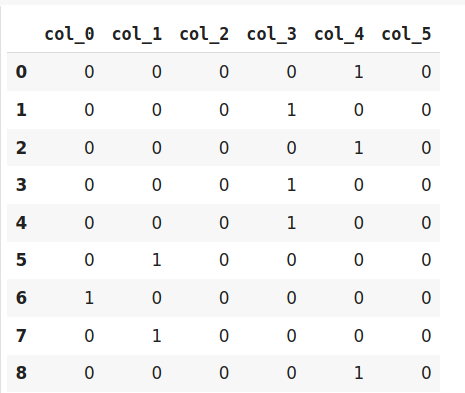### 二进制编码

#Import the libraries
import category_encoders as ce
import pandas as pd

#Create the Dataframe

#Create object for binary encoding
encoder= ce.BinaryEncoder(cols=['city'],return_df=True)

# 原始数据
data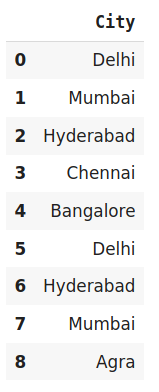# 调整和转换数据
data_encoded=encoder.fit_transform(data)
data_encoded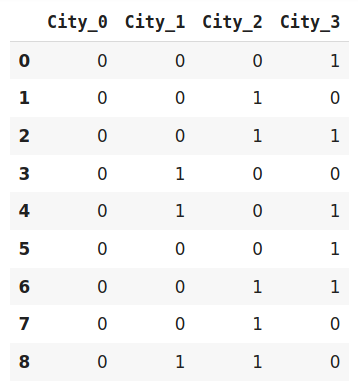### BaseN编码

#Import the libraries
import category_encoders as ce
import pandas as pd

#Create the dataframe

#Create an object for Base N Encoding
encoder= ce.BaseNEncoder(cols=['city'],return_df=True,base=5)

# 原始数据
data# 调整和转换数据
data_encoded=encoder.fit_transform(data)
data_encoded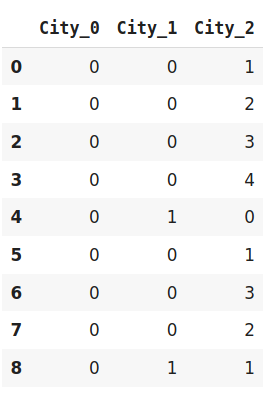### 目标编码

#import the libraries
import pandas as pd
import category_encoders as ce

#创建数据框
data=pd.DataFrame({'class':['A,','B','C','B','C','A','A','A'],'Marks':[50,30,70,80,45,97,80,68]})

#创建目标编码对象
encoder=ce.TargetEncoder(cols='class')

# 原始数据
Data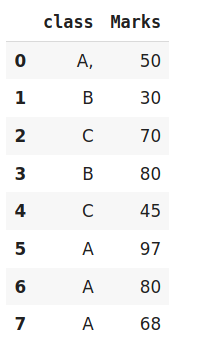# 调整并转换数据
encoder.fit_transform(data['class'],data['Marks'])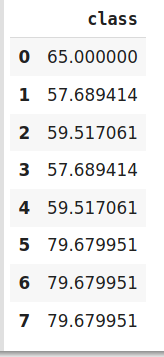1. 它可能导致目标泄漏（ target leakage）或过拟合。为了解决过拟合问题，我们可以使用不同的技术。

1. 在留一法编码中，将当前目标值从目标的整体平均值中减小以避免泄漏。
2. 在另一种方法中，我们可能会在目标统计信息中引入一些高斯噪声。这种噪声的值是模型的超参数。
2. 我们可能面临的第二个问题是训练和测试数据中类别的不正确分配。在这种情况下，类别可能采用极端值。因此，类别的目标平均值与目标的边际平均值混合在一起。

### 尾注

http://panchuang.net/

sklearn机器学习中文官方文档：
http://sklearn123.com/

http://docs.panchuang.net/